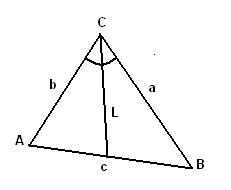homechevron_rightProfessionalchevron_rightEngineering

# Angle bisector of a triangle

This online calculator computes length of angle bisector given the lengths of triangle edgesThis online calculator computes length of angle bisector given the lengths of triangle edges (see the picture). Triangle vertices are usually named A, B and C. Triangle edges - a, b, c, where letter denotes opposite vertex. That is edge between A and B is named c, between A and C - b, between B and C - a.
The length of С's angle bisector shown on picture is:#### Angle bisector of a triangle

Digits after the decimal point: 2
Length of angle bisector

#### Similar calculatorsPLANETCALC, Angle bisector of a triangle